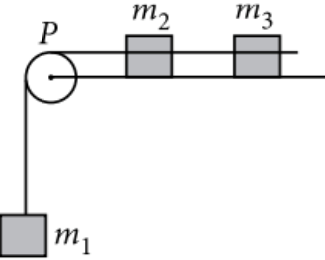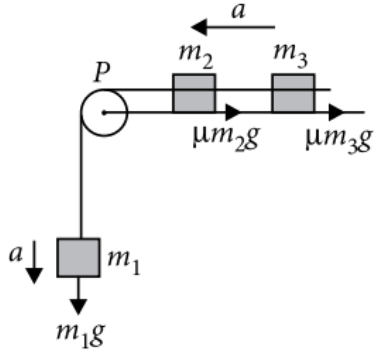Kinetic friction
Question

# A system consists of three masses   connected by a string passing over a pulley P. The mass ${m}_{1}$ hangs freely and ${m}_{2}$ and ${m}_{3}$ are on a rough horizontal table (the coefficient of friction= $\mu$)The pulley is frictionless and of negligible mass. The downward acceleration of mass ${m}_{1}$ isEasy
Solution

##Force of friction on mass ${m}_{2}=\mu {m}_{2}g$        Force of friction on mass     ${m}_{3}=\mu {m}_{3}g$        Let $\alpha$ be common acceleration of the system.

Get Instant Solutions